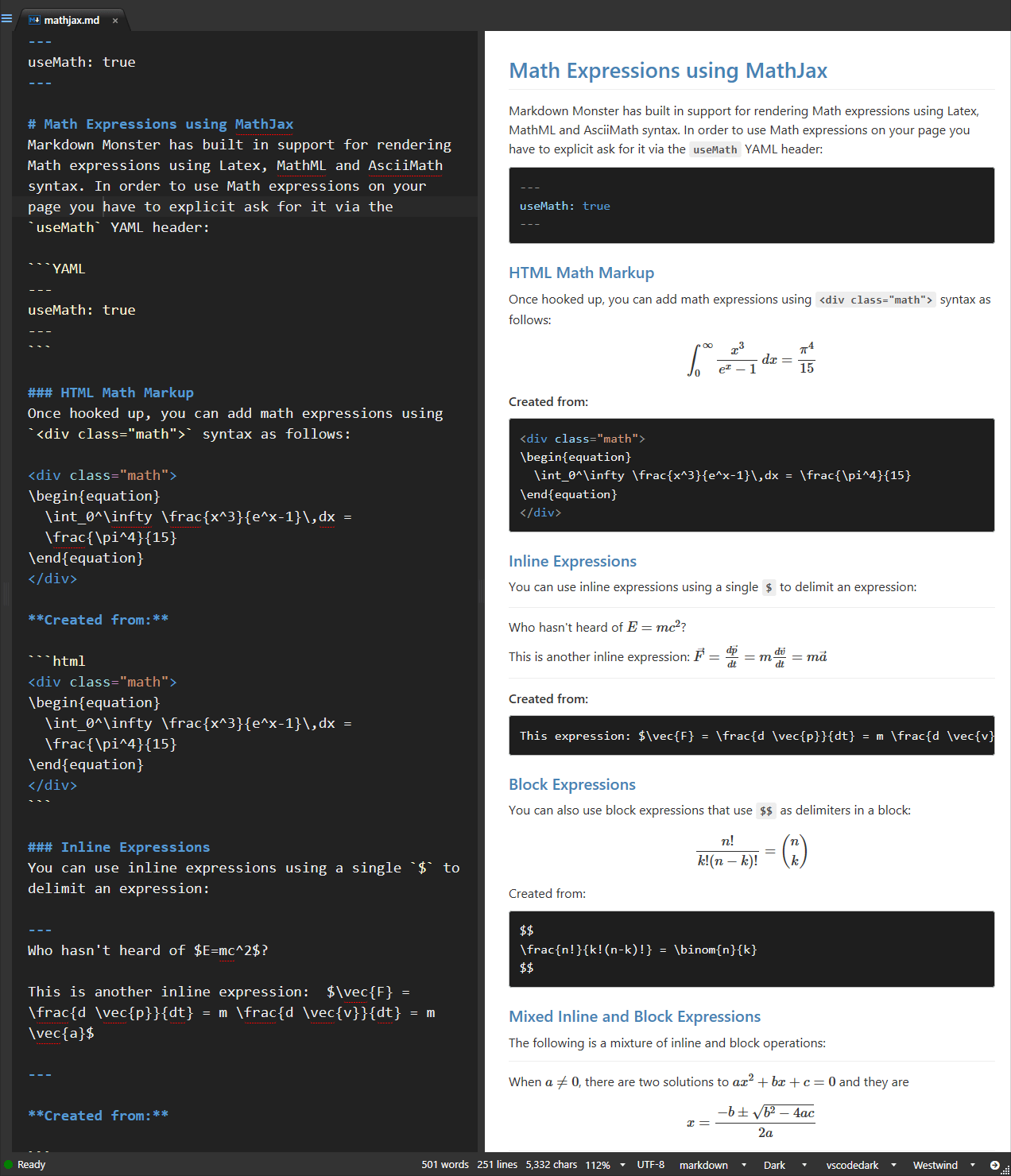#Rendering Mathematics Equations (MathTex, MML, Latex)

Math equations can be rendered in Markdown Monster and the generated HTML by using a Math processing library that is either added to the page, or to the Preview Template or your deployed site.

There are a number of libraries available and we use MathJax as an example in this topic. The output of the example below look like this:### Enabling Math Processing

Math processing is automatically enabled if you're using Math expressions in the page.

By default Math rendering is disabled as it adds some processing overhead, even when rendering non-math pages. Please ensure the following Settings are set:

• Use Mathematics on
• Allow Script Tags in Markdown (also on Edit Menu)
• Generic Attributes Off (only if there are math render issues)

If you change either of these settings you like have to reload your document or restarted MM.

#### Testing whether it Works with the Sample Math Document

You can check out a Math expression sample document in Markdown Monster from a Gist by using File -> Open From Url and copying the following URL:

https://github.com/RickStrahl/MarkdownMonster/blob/master/SampleDocuments/MathJax.md

### Embedding Math Expressions as Latex Text

You can use Latex style expressions which are the common way that Math expressions are created for HTML display. This is a bit hacky but you can specify Yaml in the header to specify you want to render Math formulas:

#### Html Markup

You can add math expressions using <div class="math"> syntax as follows:

<div class="math">
\begin{equation}
\int_0^\infty \frac{x^3}{e^x-1}\,dx = \frac{\pi^4}{15}
\end{equation}
</div>


renders as:

\begin{equation} \int_0^\infty \frac{x^3}{e^x-1}\,dx = \frac{\pi^4}{15} \end{equation}

Note that MathJax requires a top level block operation like the \begin{equation} and \end{equation} in the sample above.

You can use inline expressions using a single $ to delimit an expression: This expression:$\vec{F} = \frac{d \vec{p}}{dt} = m \frac{d \vec{v}}{dt} = m \vec{a} #### Block Expressions You can also use block expressions that use $$ as delimiters in a block: $$ \frac{n!}{k!(n-k)!} = \binom{n}{k} $$ #### Mixed Inline and Block Expressions The following is a mixture of inline and block operations: When a \ne 0, there are two solutions to ax^2 + bx + c = 0 and they are$$x = {-b \pm \sqrt{b^2-4ac} \over 2a} ### More Examples A much longer expression: <div class="math"> \begin{align} \sqrt{37} & = \sqrt{\frac{73^2-1}{12^2}} \\ & = \sqrt{\frac{73^2}{12^2}\cdot\frac{73^2-1}{73^2}} \\ & = \sqrt{\frac{73^2}{12^2}}\sqrt{\frac{73^2-1}{73^2}} \\ & = \frac{73}{12}\sqrt{1 - \frac{1}{73^2}} \\ & \approx \frac{73}{12}\left(1 - \frac{1}{2\cdot73^2}\right) \end{align} </div>  ### MathML The following is a MathML block: <math xmlns="http://www.w3.org/1998/Math/MathML" display="block"> <msup> <mrow> <mo>(</mo> <munderover> <mo>&#x2211;<!-- ? --></mo> <mrow class="MJX-TeXAtom-ORD"> <mi>k</mi> <mo>=</mo> <mn>1</mn> </mrow> <mi>n</mi> </munderover> <msub> <mi>a</mi> <mi>k</mi> </msub> <msub> <mi>b</mi> <mi>k</mi> </msub> <mo>)</mo> </mrow> <mrow class="MJX-TeXAtom-ORD"> <mspace width="negativethinmathspace" /> <mspace width="negativethinmathspace" /> <mn>2</mn> </mrow> </msup> <mo>&#x2264;<!-- = --></mo> <mrow> <mo>(</mo> <munderover> <mo>&#x2211;<!-- ? --></mo> <mrow class="MJX-TeXAtom-ORD"> <mi>k</mi> <mo>=</mo> <mn>1</mn> </mrow> <mi>n</mi> </munderover> <msubsup> <mi>a</mi> <mi>k</mi> <mn>2</mn> </msubsup> <mo>)</mo> </mrow> <mrow> <mo>(</mo> <munderover> <mo>&#x2211;<!-- ? --></mo> <mrow class="MJX-TeXAtom-ORD"> <mi>k</mi> <mo>=</mo> <mn>1</mn> </mrow> <mi>n</mi> </munderover> <msubsup> <mi>b</mi> <mi>k</mi> <mn>2</mn> </msubsup> <mo>)</mo> </mrow> [/itex]  ## Allow Script Tags is Required! In order for this to work you have to turn on "AllowRenderScriptTags": true in the Settings, so that script tags are allowed to execute in the Markdown text. If not set the script tags are mangled to disallow script processing. The script is automatically injected, but the flag has to be enabled explicitly. ## Java Script To Execute Math Expressions Markdown Monster automatically injects script into the preview HTML page to allow rendering of Math expressions and if you plan on using the output produced from this content you'll need to use these same libraries and startup code in your page(s). <script type="text/x-mathjax-config"> // enable inline parsing with single instead of / MathJax.Hub.Config({ tex2jax: { inlineMath: [['',''],['\$','\$']], displayMath: [ ['','$'], ['\$','\$'] ], processEscapes: true }, });$(document).on('previewUpdated',function() {
setTimeout(function() {
MathJax.Hub.Queue(['Typeset',MathJax.Hub,'#MainContent']);
},10);
});
</script>
<script src=""https://cdnjs.cloudflare.com/ajax/libs/mathjax/2.7.9/latest.js?config=TeX-MML-AM_CHTML"" async></script>


© West Wind Technologies, 1996-2021 • Updated: 05/24/21
Comment or report problem with topic